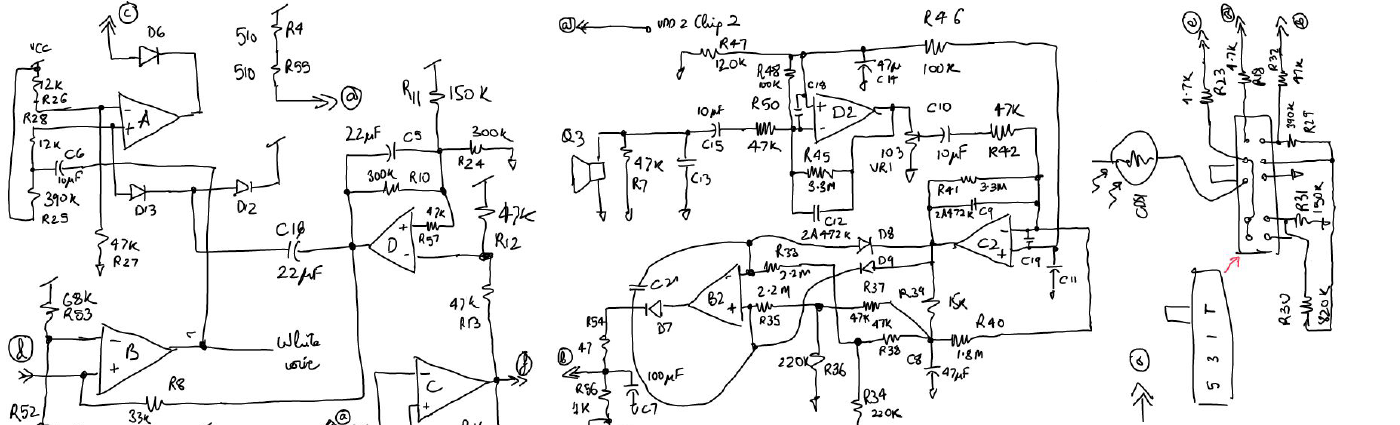# DAC Design# Specifications to Consider

1. Power Supply Rejection Ratio
2. Maximum Differential Non Linearity (DNL) : DNL = Max( | (Vout(i+1) - Vout(i)) - Videal LSB step | )
3. Maximum Integral Non Linearity (INL): INL = max0<=c<=cmax |Vout[c]-Vout-c.m| where m = (Vout[cmax]-Vout)/cmax is the slope of the line through the end points
4. Speed, Response time
5. Operating Voltage Range

# Simulations to be done

1. DNL Monte Carlo
2. INL Monte Carlo
3. PSRR for power supply extremes